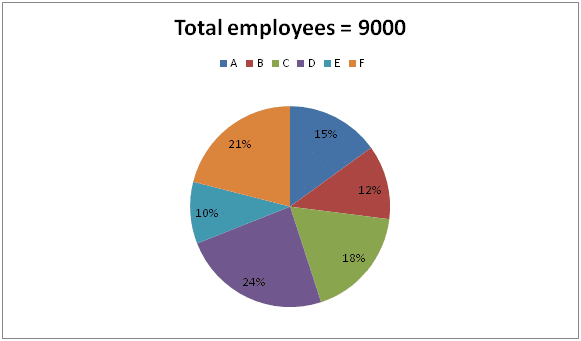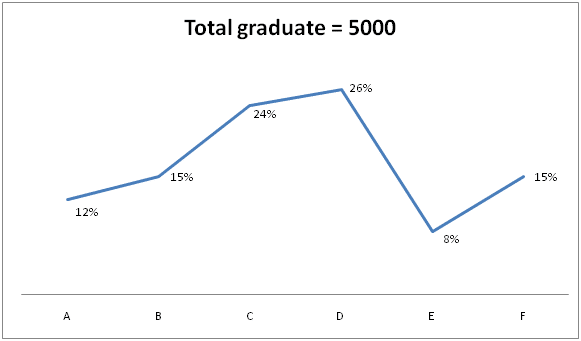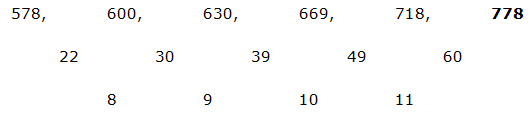# SBI Clerk Mains Quantitative Aptitude (Day-07)

Dear Aspirants, Our IBPS Guide team is providing new series of Quantitative Aptitude Questions for SBI Clerk Mains 2020 so the aspirants can practice it on a daily basis. These questions are framed by our skilled experts after understanding your needs thoroughly. Aspirants can practice these new series questions daily to familiarize with the exact exam pattern and make your preparation effective.

Start Quiz

Ensure Your Ability Before the Exam – Take SBI Clerk 2020 Mains Free Mock Test

Data Interpretation

Directions (1 – 5): Study the following information carefully and answer the given questions?

The pie chart shows the total employees working in different departments in a company and the line graph shows the percentage of graduate employees among them.1) Find the difference between the total number of graduate employees in company A to that of total number of non graduate employees in company D?

a) 340

b) 260

c) 420

d) 580

e) None of these

2) Find the average number of graduate employees in company A, B and C together?

a) 2800

b) 1950

c) 3100

d) 2550

e) None of these

3) Find the ratio between the total employees in company C to that of total non graduate employees in company E and F together?

a) 81: 82

b) 53: 57

c) 11: 13

d) 75: 79

e) None of these

4) Total employees in company D and F together is what percentage of total non graduate employees in company A and B together?

a) 250 %

b) 425 %

c) 375 %

d) 180 %

e) None of these

5) Find the difference between the average employees in company D, E and F together to that of total non graduate employees in company B, C and F together?

a) 850

b) 740

c) 930

d) 1020

e) None of these

Quantity based questions

Directions (6 – 10): Each question contains a statement followed by Quantity I, quantity II and Quantity III. Which of the following should be placed in the blank spaces of the expression “Quantity I __ Quantity II ___ Quantity III” from left to right with respect to the given statements?

6) Quantity I: (840 – 384) ÷ 24 + 787 =?

Quantity II: 45 % of 600 – 18 % of 350 =? – 62 – 252

Quantity III:  ? = (3745 + 1780) ÷ 17 + 162 + (5/11) of 880 – 15

a) >, <

b) >, >

c) <, <

d) =, >

e) None of these

7) Quantity I: 38 % of 350 + 400 % of 16 =? % of 50

Quantity II: 156 ÷ 13 + ∛19683 – (12 × 15) =? – 83

Quantity III: (√2500)3 =?

a) >, =

b) >, <

c) =, <

d) =, =

e) None of these

8) Quantity I: x2 – 17x + 72 = 0

Quantity II: y2 -19y + 88 = 0

Quantity III: 3x2 + 26x + 56 = 0

a) >, =

b) ≥, =

c) ≤, ≤

d) <, <

e) None of these

9) Quantity I: 578, 600, 630, 669, 718, ?

Quantity II: 8, 9, 14, 51, 188, ? , 5754

Quantity III: 56, 114, ? , 1384, 6925, 41556

a) <, <

b) >, >

c) =, >

d) <, >

e) None of these

10) A contains 4 pink, 6 red and 5 blue colour balls.

Quantity I: Two balls are taken randomly from the bag; find the probability of getting both is blue?

Quantity II: Two balls are taken randomly from the bag, find the probability of getting at least one pink ball

Quantity III: Two balls are taken randomly from the bag; find the probability of getting one red and one pink

a) <, >

b) >, >

c) <, <

d) =, =

e) None of these

Directions (1-5) :

The total number of graduate employees in company A

= > 5000 * (12/100) = 600

The total number of non graduate employees in company D

= > [9000 * (24/100)] – [5000 * (26/100)]

= > 2160 – 1300 = 860

Required difference = 860 – 600 = 260

The average number of graduate employees in company A, B and C together

= > 5000 * (51/100) = 2550

The total employees in company C

= > 9000 * (18/100) = 1620

The total non graduate employees in company E and F together

= > {[9000 * (10/100) – 5000 * (8/100)] + [(9000 * (21/100) – 5000 * (15/100)]}

= > {[900 – 400] + [1890 – 750]}

= > 500 + 1140 = 1640

Required ratio = 1620: 1640 = 81: 82

Total employees in company D and F together

= > 9000 * (45/100) = 4050

Total non graduate employees in company A and B together

= > {[9000 * (15/100) – 5000 * (12/100)] + [9000 * (12/100) – 5000 * (15/100)]}

= > {[1350 – 600] + [1080 – 750]}

= > 750 + 330 = 1080

Required % = (4050 / 1080) * 100 = 375 %

The average employees in company D, E and F together

= > 9000 * (55/100) * (1/3) = 1650

The total non graduate employees in company B, C and F together

= > {[9000 * (12/100) – 5000 * (15/100)] + [9000 * (18/100) – 5000 * (24/100)] + [9000 * (21/100) – 5000 * (15/100)]} * (1/3)

= > {[1080 – 750] + [1620 – 1200] + [1890 – 1140]} * (1/3)

= > (330 + 420 + 1140) / 3 = 1890 / 3

= > 630

Required difference = 1650 – 630 = 1020

Directions (6-10) :

Quantity I:

(840 – 384) / 24 + 787 = x

x = 456 / 24 + 787

x = 20 + 787 = 807 = 810

Quantity II:

45 % of 600 – 18 % of 350 = x – 62 – 252

(45/100) * 600 – (18/100) * 350 + 62 + 625 = x

x = 270 – 63 + 62 + 625

x = 894

Quantity III:

x = (3745 + 1780) ÷ 17 + 162 + (5/11) of 880 – 15

x = 5525/17 + 256 + (5/11) * 880 – 15

x = 325 + 256 + 400 – 15

x = 966

Hence, Quantity I < Quantity II < Quantity III

Quantity I:

38 % of 350 + 400 % of 16 = x % of 50

(38/100) * 350 + (400/100)*16 = (x/100) * 50

133 + 64 = x/2

197*2 = x

x = 394

Quantity II:

156 ÷ 13 + ∛19683 – (12 × 15) = x – 83

(156/13) + 27 – (12*15) + 512 = x

x = 12 + 27 – 180 + 512

x = 371

Quantity III:

(√2500)3 =?

(50)3 =?

125000 =?

Hence, Quantity I > Quantity II < Quantity III

Quantity I:

x2 – 17x + 72 = 0

(x – 8) (x – 9) = 0

x = 8, 9

Quantity II:

y2 -20y + 99 = 0

(y – 11) (y – 9) = 0

y = 11, 9

Quantity III:

3x2 + 26x + 56 = 0

3x2 + 12x + 14x + 56 = 0

3x(x + 4) + 14(x + 4) = 0

(3x + 14) (x + 4) = 0

x = -14/3, -4 = -4.667, -4

Hence, Quantity I ≤ Quantity II > Quantity III

Quantity I:The difference of difference is, 8, 9, 10, 11,..

Quantity II:

The pattern is, * 1 + 12, * 2 – 22, * 3 + 32, * 4 – 42, * 5 + 52, * 6 – 62,…

Quantity III:

The pattern is, * 2 + 2, * 3 + 3, * 4 + 4, * 5 + 5, * 6 + 6,…

Hence, Quantity I < Quantity II > Quantity III

Quantity I:

Required probability = 5C2/15C2

= (5 * 4)/(15 * 14)

= 2/21 = 0.095

Quantity II:

Required probability = 1 – (None pink)

= 1 – 11C2/15C2

= 1 – [(11 * 10)/(15 * 14)]

= 1 – 11/21 = 10/21 = 0.476

Quantity III: Two balls are taken randomly from the bag; find the probability of getting one red and one pink

Required probability = (6C1 * 4C1)/15C2

= (6 * 4)/(15 * 14)

= 4/35 = 0.114

Hence, Quantity I < Quantity II > Quantity III

 Check Here to View SBI Clerk Mains 2020 Quantitative Aptitude Questions Day 06 Day 05 Day 04 Click Here for SBI Clerk 2020 – Detailed Exam Notification